# Texas Go Math Grade 5 Lesson 7.3 Answer Key Numerical Expressions

Refer to our Texas Go Math Grade 5 Answer Key Pdf to score good marks in the exams. Test yourself by practicing the problems from Texas Go Math Grade 5 Lesson 7.3 Answer Key Numerical Expressions.

## Texas Go Math Grade 5 Lesson 7.3 Answer Key Numerical Expressions

Unlock the Problem

A numerical expression is a mathematical phrase that has numbers and operation signs but does not have an equal sign. Some numerical expressions have parentheses.

Doug went fishing for 3 days. Each day he put $15 in his pocket. At the end of each day, he had$5 left. How much money did Doug spend by the end of the trip?
• Underline the events for each day.
• Circle the number of days these events happened.

Which expression matches the meaning of the words?

Think: Each day he took $15 and had$5 left. He did this for 3 days.
($15 –$5) ← Think: What expression can you write to show how much money Doug spends in one day?
3 × ($15 –$5) ← Think: What expression can you write to show how much money Doug spends in three days?The expression matches the meaning of the words is 3 × ($15 –$5).
Explanation:
Doug went fishing for 3 days. Each day he put $15 in his pocket. At the end of each day, he had$5 left. In one day Doug spend ($15 –$5). By the end of the trip Doug spend 3 x ($15 –$5). The expression matches the meaning of the words is 3 × ($15 –$5). Doug spend $30 by the end of the trip. Math Talk Mathematical Processes Explain how the expression of what Doug spent in three days compares to the expression of what he spent in one day. Answer: Example Which problem matches the expression$20 – ($12 +$3)?

Kim has $20 to spend for her fishing trip. She spends$12 on a fishing pole. Then she finds $3. How much money does Kim have now? List the events in order. First: Kim has$20.
Next: ________________
Then: ________________
Do these words match the expression? ____________
First: Kim has $20. Next:$20 – $12 Then: ($20 – $12) +$3
Do these words match the expression? No
Explanation:
Kim has $20 to spend for her fishing trip. She spends$12 on a fishing pole. The expression is ($20 –$12). Then she finds $3. Now the expression is ($20 – $12) +$3. Now Kim have $11. These words does not matches with the expression$20 – ($12 +$3).
Kim has $20 to spend for her fishing trip. She spends$12 on a fishing pole and $3 on bait. How much money does Kim have now? List the events in order. First: Kim has$20.
Next: ________________
Then: ________________
Do these words match the expression? ____________
First: Kim has $20. Next:$20 – $12 Then:$20 – ($12 +$3)
Do these words match the expression? Yes
Explanation:
Kim has $20 to spend for her fishing trip. She spends$12 on a fishing pole and $3 on bait. The expression is$20 – ($12 +$3). These words matches the given expression $20 – ($12 + $3). Now Kim have$5.

Share and Show

Circle the expression that matches the words.

Question 1.
Teri had 18 worms. She gave 4 worms to Susie and 3 worms to Jamie.
(18 – 4) + 3
18 – (4 + 3)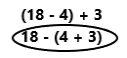Explanation:
Teri had 18 worms. She gave 4 worms to Susie and 3 worms to Jamie. The expression that matches the words is
18 – (4 + 3). So draw a circle to this expression 18 – (4 + 3).

Rick had $8. He then worked 4 hours for$5 each hour.
$8 + (4 ×$5)
($8 + 4) ×$5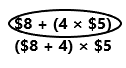Explanation:
Rick had $8. He then worked 4 hours for$5 each hour. The expression that matches the words is $8 + (4 ×$5). So draw a circle to this expression $8 + (4 ×$5).

Write an expression to match the words.

Question 3.
Greg walks dogs for 5 days. He walks 4 dogs each morning and 3 dogs each afternoon.
5 x (4 + 3)
Explanation:
Greg walks dogs for 5 days. He walks 4 dogs each morning and 3 dogs each afternoon. The word that matches the expression is 5 x (4 + 3).

Question 4.
Lynda has 27 fewer fish than Jack. Jack has 80 fish.
80 – 27
Explanation:
Lynda has 27 fewer fish than Jack. Jack has 80 fish. The words that match the expression are 80 – 27.

Write words to match the expression.

Question 5.
34 – 17
Varun had 34 cookies and he gave 17 of them to his friend.
Explanation:
Varun had 34 cookies and he gave 17 of them to his friend. These words match the given expression 34 – 17.

Question 6.
6 × (12 – 4)
For the last 6 years, Susie got $12 for her birthday and gave$ 4 of that to her little brother.
Explanation:
For the last 6 years, Susie got $12 for her birthday and gave$ 4 of that to her little brother. These words matches the given expression 6 x (12 – 4).

Problem Solving

Write an expression to match the words.

Question 7.
Jose has 9 pens. He buys 3 more. He shares all the pens equally among 6 friends.
(9 + 3) ÷ 6
Explanation:
Jose has 9 pens. He buys 3 more. He shares all the pens equally among 6 friends. These words match with this expression (9 + 3) ÷ 6.

Go Math Lesson 7.3 Answer Key 5th Grade Math Expressions Question 8.
Monique had $20. She spent$5 on lunch and $10 at the bookstore. Answer:$20 – ($5 +$10)
Explanation:
Monique had $20. She spent$5 on lunch and $10 at the bookstore. These words match with this expression$20 – ($5 +$10).

Write words to match the expression.

Question 9.
($8 +$14) – (4 × $5) Answer: Jasmine has$8 and her husband gave $14. She bought 4 chocolates which were$5 each. These words match with the expression ($8 +$14) – (4 × $5). Question 10. (12 × 6) – (3 × 2) Answer: In one year, Pooja will get 6 balloons for every month. She gave two balloons each to her three brothers. These words match with the expression (12 × 6) – (3 × 2). Connect Draw a line to match the expression with the words. Question 11.Answer: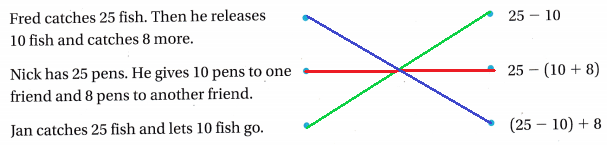Explanation: Fred catches 25 fish. Then he releases 10 fish and catches 8 more. The expression is (25 – 10) + 8. Draw a line from these words to the expression (25 – 10) + 8. Nick has 25 pens. He gives 10 pens to one friend and 8 pens to another friend. The expression is 25 – (10 + 8). Draw a line from these words to the expression 25 – (10 + 8). Jan catches 25 fish and lets 10 fish go. The expression is 25 – 10. Draw a line from these words to the expression 25 – 10. Problem-Solving Use the rule and the table for 12-13.Go Math Answer Key Grade 5 Lesson 7.3 Question 12. Represent Write a numerical expression to represent the total number of lemon tetras that could be in a 20-gallon aquarium. Answer: 20 ÷ 2 The total number of tetras in 20-gallon of aquarium is 10. Explanation: The fish in a aquarium allows 1 gallon of water for each inch of length. The lemon tetra is 2 inches length. The numerical expression 10 x 2 represents the total number of lemon tetras that could be in a 20-gallon aquarium. The total number of tetras in 20-gallon of aquarium is 10. Question 13. H.O.T. There are tiger barbs in a 15-gallon aquarium and giant danios in a 30-gallon aquarium. Write a numerical expression to represent the total number of fish that could be in both aquariums.Answer: (15 ÷ 3) + (30 ÷ 5) The above numerical expression represents the total number of fish in both aquariums. Explanation: The fish in a aquarium allows 1 gallon of water for each inch of length. There are tiger barbs in a 15-gallon aquarium and giant danios in a 30-gallon aquarium. The tiger barbs is 3 inches length. The giant danios is 5 inches length. The numerical expression (15 ÷ 3) + (30 ÷ 5) represent the total number of fish in both aquariums. Question 14. Write a word problem for an expression that is three times as great as (15 + 7). Then write the expression. Answer: The three brothers Ram, Rahim, Robert each have 15 mangoes and 7 cherries with them. The total number of fruits altogether they have is 3 x (15 + 7). The expression is 3 x (15 + 7). Question 15. What’s the Question? Lu has 3 swordtails in her aquarium. She buys 2 more swordtails. Answer: How many gallons of water is needed in an aquarium when Lu buys two more swordtails along with the three swordtails already he have. Question 16. Multi-Step Tammy has 26 stamps. She buys 19 more stamps. She shares all the stamps equally among 9 friends. Write an expression to match the words. How many stamps does each friend get? Answer: The expression matches the words (26 + 19) ÷ 9 (45) ÷ 9 5 Each friend gets 5 stamps. Explanation: Tammy has 26 stamps. She buys 19 more stamps. She shares all the stamps equally among 9 friends. The expression that matches the words is (26 + 19) ÷ 9. Add 26 stamps with 19 stamps the sum is 45 stamps. Divide 45 stamps by 9 friends the result is 5 stamps. Each friend gets 5 stamps. Daily Assessment Task Fill in the bubble completely to show your answer. Lesson 7.3 Answer Key 5th Grade Go Math Question 17. Daniel started a video game with 30 tokens. He earned 8 more tokens for jumping on a star but lost 6 tokens for falling into a hole. When he passed a checkpoint, his number of tokens doubled! Then he spent 20 tokens to buy a flashlight. Which expression shows how many tokens he has left? (A) 2 × (30 + 8 – 6 – 20) (B) 2 × (30 + 8) – (20 + 6) (C) 2 × 30 + 8- 6- 20 (D) 2 × (30 + 8 – 6) – 20 Answer: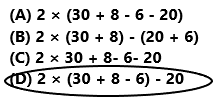The expression 2 x (30 + 8 – 6) – 20 shows the token left with him. So, option D is correct. Explanation: Daniel started a video game with 30 tokens. He earned 8 more tokens for jumping on a star, but lost 6 tokens for falling into a hole. The expression is (30 + 8 – 6). When he passed a checkpoint, his number of tokens is doubled. Then he spent 20 tokens to buy a flashlight. The expression 2 x (30 + 8 – 6) – 20 shows the token left with him. Question 18. Enrique brought$30 to the mall and spent $14 on a soccer ball and$6 on lunch. Which expression represents the situation?
(A) 30 ÷ (14 – 6)
(B) 30 – (14 + 6)
(C) 30 ÷ (14 – 6)
(D) 30 – 14 + 30 – 6The expression is 30 – (14 + 6).
So, option B is correct.
Explanation:
Enrique brought $30 to the mall and spent$14 on a soccer ball and $6 on lunch. The expression 30 – (14 + 6) represents the situation. Question 19. Multi-Step Terrel has 8 nickels. Heather has twice as many nickels as Terrel. Heather spends 4 nickels to buy a pencil. Each nickel is worth 5 cents. Which expression shows how many cents Heather has left? (A) 5 + 2 + 8 – 4 (B) 5 × (2 × 8) – 4 (C) 5 × (2 × 8 – 4) (D) 5 × 2 + 8 – 4 Answer: Texas Test Prep Math Expressions 5th Grade Answer Key Lesson 7.3 Question 20. Josh has 3 fish in each of the 5 buckets. Then he releases 4 fish. Which expression matches the words? (A) (3 × 4) – 5 (B) (5 × 4) – 3 (C) (5 × 3) – 4 (D) (5 – 3) × 4 Answer: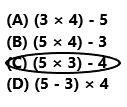The expression is (5 x 3) – 4. So, option C is correct. Explanation: Josh has 3 fish in each of 5 buckets. Then he releases 4 fish. The expression that matches the words is (5 x 3) – 4. ### Texas Go Math Grade 5 Lesson 7.3 Homework and Practice Answer Key Write an expression to match the words. Question 1. Frank had$25 in his checking account. He withdrew $15 cash and$9 to pay a bill.
$25 – ($15 + $9) Explanation: Frank had$25 in his checking account. He withdrew $15 cash and$9 to pay a bill. The expression that matches the words is $25 – ($15 + $9). Question 2. Patricia charges$12 to rake the yard. She charges $5 per hour to bag the piles of leaves for 2 hours. Answer:$12 + ($5 x 2) Explanation: Patricia charges$12 to rake the yard. She charges $5 per hour to bag the piles of leaves for 2 hours. The expression that matches the words is$12 + ($5 x 2). Write words to match the expressions. Question 3. (5 × 2) × (3 × 15) Answer: Each person in the five couples are holding three sets of fifteen pens each. These words matches the expression (5 × 2) × (3 × 15). Go Math 5th Grade Practice and Homework Lesson 7.3 Answer Key Question 4. 36 + (2 × 3) Answer: Rohan has 36 coins with him. His two brothers gave extra three coins each. These words matches the expression 36 + (2 × 3). Question 5. (5 + 10) ÷ 5 Answer: Raj decided to distribute five chocolates and ten fruits to his five best friends equally. These words matches the expression (5 + 10) ÷ 5. Question 6. (12 × 2) + (4 × 3) Answer: In Joe’s apartment, surprisingly twelve couples have twins and four couples have triplets. These words matches the expression (12 × 2) + (4 × 3). Problem-Solving Question 7. Dominick makes a design with 5 rows of 6 tiles. He frames the same design in 7 different frames to sell at the craft fair. Write an expression to match the words. How many tiles does Dominick use? Answer: Go Math Grade 5 Chapter 7 Lesson 7.3 Answer Key Question 8. Tim buys three 6-packs of apple juice boxes and five 4-packs of cranberry juice boxes for his party. Write an expression to match the words. How many boxes of juice does Tim buy? Answer: The expression that matches the words is (3 x 6) + (5 x 4). (3 x 6) + (5 x 4) 18 + 20 38 Tim buys 38 boxes of juice. Explanation: Tim buys three 6-packs of apple juice boxes and five 4-packs of cranberry juice boxes for his party. The expression that matches the words is (3 x 6) + (5 x 4). Tim buys 38 boxes of juice. Lesson Check Fill in the bubble completely to show your answer. Use the price list for 9-11.Question 9. Which expression shows the cost of one adult admission ticket and 9 thrill ride tickets? (A)$15 + (9 × $3) (B)$12 + (9 × $3) (C)$15 + (9 + $3) (D) ($15 × 9) + $3 Answer:So, option A is correct. Explanation: The above table is the ultimate amusement park. In that, we can observe tickets and prices. The cost of one adult admission ticket is$15. For each person, the thrill ride ticket is $3. Multiply 9 with$3. The expression is $15 + (9 ×$3) shows the cost of one adult admission ticket and 9 thrill ride tickets.

Bandar and Courtney each paid $12 for admission. They bought 14 thrill-ride tickets. Which expression shows their cost? (A)$12 + (14 × $3) (B) (2 ×$12) + (14 × $3) (C) (2 ×$12) + 14
(D) $12 + (14 +$3)Option B is correct.
Explanation:
Bandar and Courtney each paid $12 for admission. They bought 14 thrill-ride tickets. For each person, the thrill ride ticket is$3as we can observe in the above table. The expression (2 × $12) + (14 ×$3) shows their cost.

Question 11.
Riley bought a child admission ticket and 6 fun land ride tickets. He used a coupon for a $5 discount. Which expression shows Riley’s total cost? (A)$12 + (6 × $2) (B)$12 + ($6 +$2) – $5 (C)$12 + (6 × $2) –$5
(D) $12 + (6 ×$2) + $5 Answer: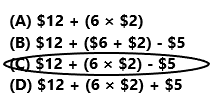Riley’s total cost is$12 + (6 × $2) –$5.
So, option C is correct.
Explanation:
Riley bought a child admission ticket and 6 fun land ride tickets. The cost of a child admission ticket is $12 and each person the thrill ride ticket is$3 as we can observe in the above table. He used a coupon for a $5 discount. The expression$12 + (6 × $2) –$5 shows the Riley’s total cost.

Multi-Step The classroom has 12 chairs on the right side of the room, 15 chairs on the left, and 9 chairs stacked in the back. Mrs. Kim wants to divide the chairs evenly into 3 groups. Which expression represents the situation?
(A) 12 + 15 + 9 × 3
(B) 12 + 15 + (9 ÷ 3)
(C) 12 + 15 + 9 + 3
(D) (12 + 15 + 9) ÷ 3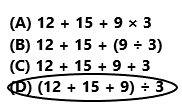The expression is (12 + 15 + 9) ÷ 3.
So, option D is correct.
Explanation:
The classroom has 12 chairs on the right side of the room, 15 chairs on the left, and 9 chairs stacked in the back. Mrs. Kim divides the chairs evenly into 3 groups. The expression represents the situation is (12 + 15 + 9) ÷ 3.

Question 13.
Multi-Step Mr. Richardson is driving to a town 85 miles away. He drives 35 miles per hour for 2 hours before stopping for lunch. He drives 6 more miles and stops for gas. Which expression shows how many miles Mr. Richardson has left to drive?
(A) 85 – (35 × 2) – 6
(B) 85 – 35 – 2 -6
(C) (85 – 35) × 2 – 6
(D) 85 – 35 + 2 -6